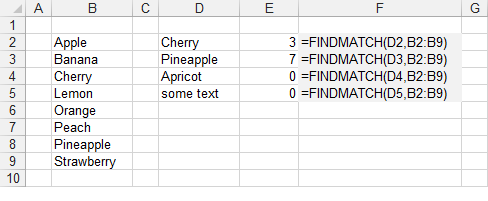# FINDMATCH

Returns the position of an item in a list.
For instructions on how to add a function to a workbook refer to the page under Inserting Functions

`'sLookupValue - The value you want to find.'rgeLookupArray - The contiguous range of cells containing possible lookup values.Public Function FINDMATCH(ByVal sLookupValue As String, _                           ByVal rgeLookupArray As Range) As Integer                            Dim ilow As Integer Dim ihigh As Integer Dim imiddle As Integer    ilow = 0    ihigh = rgeLookupArray.Cells.Count                               Do While ilow <= ihigh       imiddle = (ilow + ihigh) / 2             If VBA.StrComp(sLookupValue, rgeLookupArray.Cells(imiddle, 1).Value, _                      VbCompareMethod.vbTextCompare) = 0 Then          FINDMATCH = imiddle          Exit Function       End If          If VBA.StrComp(sLookupValue, rgeLookupArray.Cells(imiddle, 1).Value, _                      VbCompareMethod.vbTextCompare) = -1 Then          ihigh = imiddle - 1       Else          ilow = imiddle + 1       End If    Loop       Call MsgBox("This entry has not been found")    FINDMATCH = 0 End Function `

The "rgeLookupArray" must be a single block of cells, either in a row or a column.
This function uses a binary search and is very similar to the MATCH function.